Function Repository Resource:

# SequenceToProduct

Turn a sequence of expressions into a symbolic product

Contributed by: Wolfram|Alpha Math Team
 ResourceFunction["SequenceToProduct"][{e1,e2,…},n] attempts to return an inactive product representing e1×e2×… where the user-supplied variable n is used in forming the generic term.

## Details

If successful, ResourceFunction["SequenceToProduct"] returns an expression with head . Using Activate on this output will attempt to evaluate the product.
ResourceFunction["SequenceToProduct"] gives special treatment to the global symbol …, representing a sequence of ellided expressions. The ellipsis symbol … can be input with the keyboard shortcut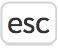.

## Examples

### Basic Examples (10)

Convert a simple finite sequence to a product:

 In:=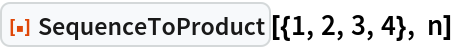Out=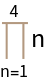Convert an elided sequence to a product:

 In:=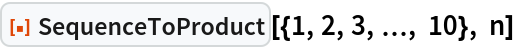Out=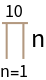Evaluate with Activate:

 In:=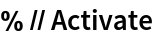Out=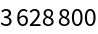Convert an infinite sequence to a product:

 In:=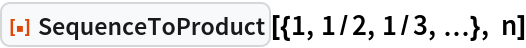Out=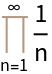Convert an geometric sequence to a product:

 In:=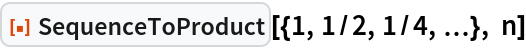Out=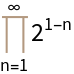Convert another geometric sequence to a product and evaluate:

 In:=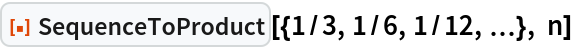Out=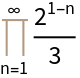In:=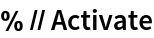Out=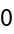Convert an arithmetic sequence to a product:

 In:=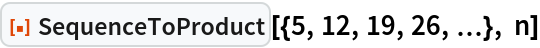Out=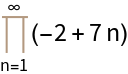Convert another arithmetic sequence to a product and evaluate:

 In:=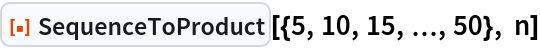Out=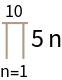In:=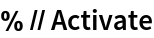Out=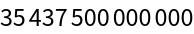Convert an alternating sequence to a product and evaluate:

 In:=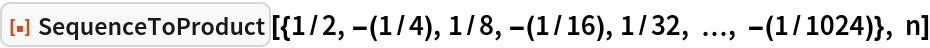Out=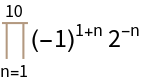In:=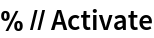Out=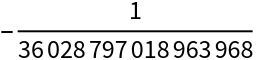Convert a rational sequence to a product:

 In:=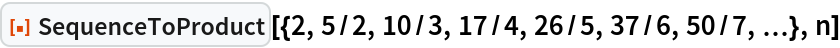Out=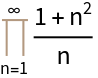Convert a hypergeometric sequence to a product:

 In:=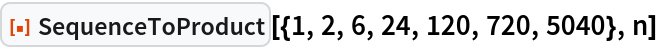Out=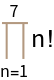In:=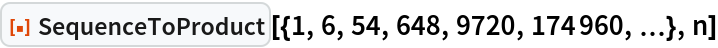Out=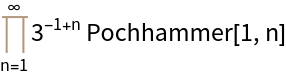### Scope (1)

The ellipsis symbol (…) can appear anywhere within the input sequence:

 In:=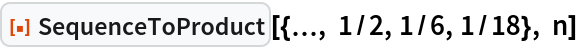Out=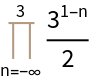In:=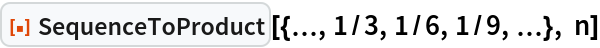Out=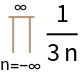### Properties and Relations (3)

If successful, SequenceToProduct returns an expression with head :

 In:=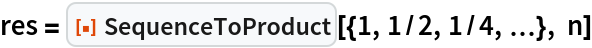Out=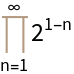In:=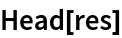Out=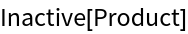If unable to infer the elided part of a sequence, SequenceToProduct will return unevaluated:

 In:=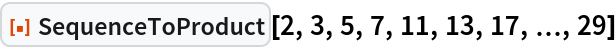Out=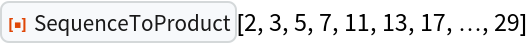SequenceToProduct uses FindSequenceFunction to recognize patterns in the input sequence:

 In:=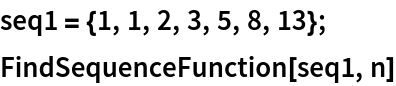Out=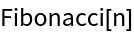In:=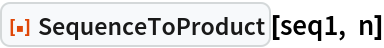Out=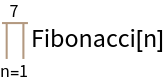In:=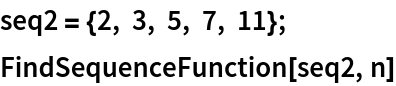Out=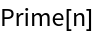In:=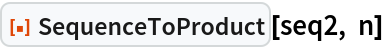Out=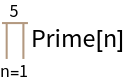## Publisher

Wolfram|Alpha Math Team

## Version History

• 3.0.0 – 23 March 2023
• 2.1.0 – 20 May 2021
• 2.0.0 – 24 January 2020
• 1.0.0 – 16 October 2019